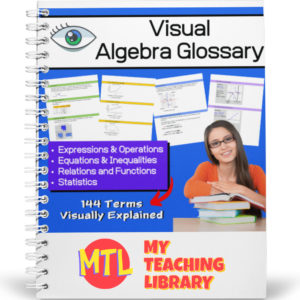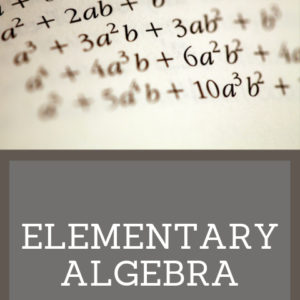Showing all 5 results

•\$15.00

Give your Algebra I and Algebra II students a tool they can really use! This Visual Algebra Glossary will be an invaluable resource giving them explanations, definitions and examples of 144 terms. Easy to follow and understand, students will turn to this resource while learning new concepts, completing work and studying for exams.

There are four color coded sections, each with an term index:

• – Expressions & Operations
• – Equations & Inequalities
• – Relations & Functions
• – Statistics

73 pages

•\$10.00

Elementary Algebra is designed to meet the scope and sequence requirements of a a full year of elementary algebra. Each topic builds upon previously developed material to demonstrate the cohesiveness and structure of mathematics. Topics studied:

Arithmetic Review
Real Numbers
Algebraic Expressions & Equations
Linear Equations & Inequalities
Polynomials
Graphing Linear Equations & Inequalities
Rational Expressions
Roots, Radicals and Square Root Equations
Systems of Linear Equations

•\$3.50

Written in 1895, this text includes over 1000 exercises for practice. Topics covered include operations, factoring, fractions, complex fractions, solving equations, and solving simultaneous equations. Answers are provided at the end of the book.

•\$9.99

This algebra textbook teaches students the following:

• Pre-Algebra
• Solving Linear Equations
• Graphing
• Inequalities
• Systems of Equations
• Polynomials
• Factoring
• Rational Expressions
•